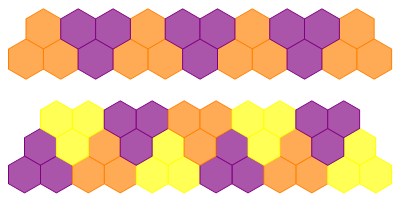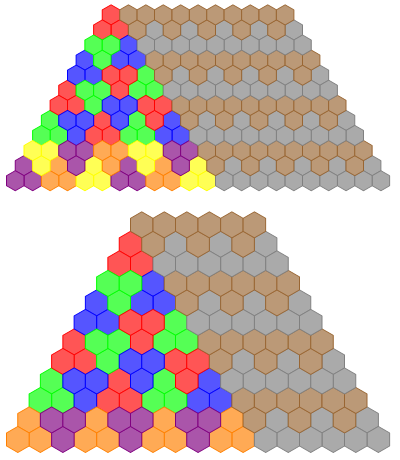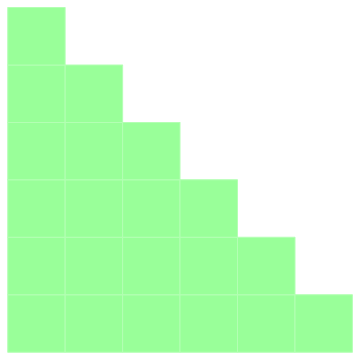# The world still exists

It transpires that the world didn’t actually end yesterday. At the very least, Descartes’ famous deduction ‘cogito ergo sum’ seems to imply that. To summarise, the Mayan calendar has finished its 13th long count cycle; equivalently, 13×20×20×18×20 days have passed since the beginning of the aforementioned calendar. Suffice it to say, it was rather anticlimatic, with nothing particularly interesting happening.

Depicted below is a tiling of a triangular arrangement of hexagons of side length s = 9 with trihexes. An interesting problem, solved by John Conway, asks which side lengths can actually be tiled with trihexes.Obviously, it can be done in the cases s = 2 and s = 9, and it’s vacuously true for s = 0. Can we do it for other sizes? Yes, we can attain 11 and 12 by augmenting the previous tiling:You can also add multiples of 12 rows by the following scheme, thereby attaining any side lengths congruent to {0, 2, 9, 11} mod 12.It’s trivially obvious that you can’t tile a triangle with side length congruent to {1, 4, 7, 10} mod 12, since the number of hexagons is not divisible by 3. This leaves the cases of {3, 5, 6, 8} mod 12, which are impossible for more subtle reasons. We can convert this problem into one on a square grid by applying an affine transformation:Can we tile this with L-trominos in two orientations? If we consider the perimeter of the region to be a group word (where a = up, b = left, a’ = down, b’ = right), then the two types of tromino are equivalent to the relations bbaa = abab and aabb = baba. Under these relations, the perimeter (which reduces to bbbaaab’a’b’a’b’a’) is not equivalent to the identity, so we can’t tile the entire area with trominos. This can be proved by constructing a group where the relations bbaa = abab and aabb = baba hold, but where bbbaaa ≠ ababab. Some example groups are mentioned here.

This entry was posted in Uncategorized. Bookmark the permalink.